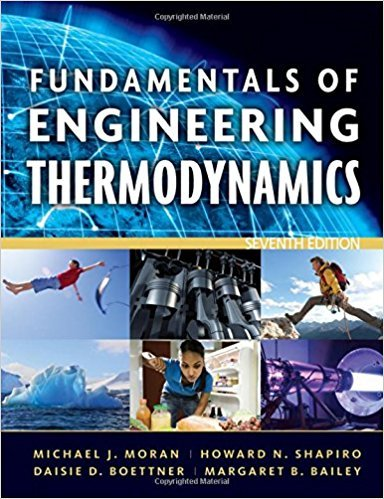×
Get Full Access to Fundamentals Of Engineering Thermodynamics - 7 Edition - Chapter 4 - Problem 84p
Get Full Access to Fundamentals Of Engineering Thermodynamics - 7 Edition - Chapter 4 - Problem 84p

×

# Energy Analysis of Control Volumes at Steady | Ch 4 - 84PISBN: 9780470495902 50

## Solution for problem 84P Chapter 4

Fundamentals of Engineering Thermodynamics | 7th Edition

• Textbook Solutions
• 2901 Step-by-step solutions solved by professors and subject experts
• Get 24/7 help from StudySoup virtual teaching assistantsFundamentals of Engineering Thermodynamics | 7th Edition

4 5 1 296 Reviews
31
1
Problem 84P

Problem 84P

Energy Analysis of Control Volumes at Steady State

Figure P4.84 provides steady-state data for the ducting ahead of the chiller coils in an air conditioning system. Outside air at 90°F is mixed with return air at 75°F. Stray heat transfer is negligible, kinetic and potential energy effects can be ignored, and the pressure throughout is 1 atm. Modeling the air as an ideal gas with cp = 0.24 Btu/lb · R, determine (a) the mixed-air temperature, in °F, and (b) the diameter of the mixed-air duct, in ft.Step-by-Step Solution:

Solution 84P

Step 1 of 5:

In this question, we need to find temperature of mixed air inIn part b, we need to find the diameter of the mixed air duct inData given

Temperature of outside airTemperature of mixed airPressureSpecific pressurePart a:

Step 2 of 5

Step 3 of 5

##### ISBN: 9780470495902

The full step-by-step solution to problem: 84P from chapter: 4 was answered by , our top Engineering and Tech solution expert on 07/20/17, 09:01AM. Fundamentals of Engineering Thermodynamics was written by and is associated to the ISBN: 9780470495902. The answer to “Energy Analysis of Control Volumes at Steady StateFigure P4.84 provides steady-state data for the ducting ahead of the chiller coils in an air conditioning system. Outside air at 90°F is mixed with return air at 75°F. Stray heat transfer is negligible, kinetic and potential energy effects can be ignored, and the pressure throughout is 1 atm. Modeling the air as an ideal gas with cp = 0.24 Btu/lb · R, determine (a) the mixed-air temperature, in °F, and (b) the diameter of the mixed-air duct, in ft.” is broken down into a number of easy to follow steps, and 87 words. Since the solution to 84P from 4 chapter was answered, more than 1117 students have viewed the full step-by-step answer. This textbook survival guide was created for the textbook: Fundamentals of Engineering Thermodynamics, edition: 7. This full solution covers the following key subjects: air, Mixed, state, steady, Energy. This expansive textbook survival guide covers 14 chapters, and 1501 solutions.

Unlock Textbook Solution